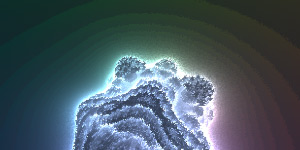# HYPERSONIC MANDELBULB

732 words ~ 3-7 minsMathieu 'p01' Henri on March 6th, 2013

# Hypersonic Mandelbulb

A hasty invalid JS1k entry using webGL+Audio. Possibly the first one.## That was quick.

JS1k started in February, and Steven Wittens made a pretty neat Jelly Mandelbox using webGL in 1.1k. Of course this gem was off contest due to its size and use of webGL but it got me wondering about the potential of webGL within the constraints of JS1k.

The Mandelbulb craze is over but it remains a nice fractal and the GLSL sandbox 6601 was floating around Twitter recently. The code was clean but unfortunately without comment as to who wrote it.

Anyways, applying the usual optimizations: one letter variables, inlining everything possible, flipping some tests around, etc.. brought the fragment shader down to ~520 bytes in no time. Then I plugged the shader into the webGL setup and main loop of Micro Nova, added some glitches to the position vector, a pinch of sound using the simple setup of JS1k SpeechSynthesizer and a Matraka-esque melody, played a bit with the colors, sent the whole thing went through First Crush by @tpdown and reached 1024 byte packed.

And voila! Within a few hours, Hypersonic Mandelbulb was born and weighed 1081 bytes unpacked including:

• 29 bytes of HTML + CSS
• 558 bytes of GLSL ( 29 and 514 for the vertex and fragment shader respectively + 15 bytes in common )
• 494 bytes of JavaScript including 41 bytes for the main loop and 188 for the Audio.

It felt pretty good at the time and ended in a JSbin.

## Not so fast!

While the initial result was, AFAIK, the first 1k intro featuring webGL+Audio and didn't look or sound too bad, I was unhappy with to fall so close to 1k. Surely those damn 57 bytes could be optimized away. So I looked at the source code again.

Quickly glaring mistakes and optimizations appeared. Some constant vectors and floats could be avoided, variables could be re-used, the music synthesizer could be simplified a bit. In the process I managed to improve the colors, glithces and make the level of details depend on the distance to the camera.

Eventually the whole source ended at 1020 bytes!

• 29 bytes of HTML + CSS
• 508 bytes of GLSL ( 29 and 464 for the vertex and fragment shader respectively + 15 bytes in common )
• 483 bytes of JavaScript including 41 bytes for the main loop and 175 for the Audio.

No need for a packer anymore, and we can leave the setup of JS1k and make a standalone version in 1021 bytes.

## Source codes

The code shouldn't be totally insanity inducing to read. The various parts ( HTML setup, methods hashing, webGL setup + shaders, Audio setup and synth, main loop ) and optimizations should be easy to spot with two versions of the code plus the original shader.

``````// Initial release - 1081 bytes unpacked
b.innerHTML='<canvas id=d style=width:99%>';for(x in g=d.getContext('experimental-webgl'))g[x[t=0]+x]=g[x];with(g){for(p=cP(q=' t;void main(){');s=cS(t+35632);ce(s),aS(p,s))sS(s,t++?'attribute vec4'+q+'gl_Position=t;}':'uniform lowp float'+q+'lowp vec2 P=gl_FragCoord.xy/75.-vec2(2.+mod(tan(gl_FragCoord.y+t),.02),.5);lowp vec3 p=vec3(sin(t),cos(t),2.+sin(t)),g=normalize(-p),z=vec3(.0,1.,.0),F=normalize(cross(g,z)*P.x+z*P.y+g*1.5),v;lowp float e=1.,s,u,r,d,C=0.;for(int i=0;i<99;++i){if(e>.001&&C<4.){v=p;d=1.;for(int n=0;n<9;++n){r=length(v);if(r<4.)d=pow(r,6.)*7.*d+1.,v=vec3(sin(s=7.*acos(v.z/r))*cos(u=7.*atan(v.y,v.x)),sin(u)*sin(s),cos(s))*pow(r,7.)+p;}e=.5*log(r)*r/d;C+=e;p+=F*e;gl_FragColor+=vec4(vec3(.01,.02,.03)+p*.01,1.);}}}');r='data:audio/wav;base64,UklGRl9vT19XQVZFZm10IBAAAAABAAEAQB8AAEAfAAABAAgA';for(i=0;++i<1e5;)q+='10145013202140203412'[(i>>10&15)+(i>>12&4)]*i*.1&9;o=new Audio(r+btoa(r+q));o.play(setInterval('g.dr(4,g.uniform1f(g.gf(p,"t"),t+=.01),3)',o.loop=vA(eV(bf(k=34962,cB())),2,5126,lo(p),ug(p),bD(k,new Float32Array([1,1,1,-3,-3,1]),k+82))|33))}``````
``````// Final release - 1020 bytes
b.innerHTML='<canvas id=d style=width:99%>';for(x in g=d.getContext('experimental-webgl'))g[x[t=0]+x]=g[x];with(g){for(p=cP(q=' t;void main(){');s=cS(t+35632);ce(s),aS(p,s))sS(s,t++?'attribute vec4'+q+'gl_Position=t;}':'uniform '+(w='lowp float')+q+w+' C,u,r=sin(t),s,e=1.5,d;lowp vec3 P=gl_FragCoord.xyz/75.+mod(length(gl_FragCoord)*t,.03)-2.,p=vec3(r,cos(t),e+r),F=normalize(vec3(p.z*P.x,(P.y+e)*length(p),-p.x*P.x)-p*e),v;for(int i=0;i<99;++i)if(e>C*.001&&C<4.){v=p;d=1.;for(int n=0;n<6;++n){r=length(v);if(r<4.)d=pow(r,5.)*6.*d+1.,e=sin(s=6.*acos(v.z/r)),v=vec3(e*cos(u=6.*atan(v.y,v.x)),e*sin(u),cos(s))*pow(r,6.)+p;}C+=e=.5*log(r)*r/d;p+=F*e;gl_FragColor+=vec4(vec3(p-F+2.)*.01,d);}}');for(r='data:audio/wav;base64,UklGRl9vT19XQVZFZm10IBAAAAABAAEAQB8AAEAfAAABAAgA';t<153;t+=.001)q+='1014501320214020'[t%16^(t/4&4)]*t*42&9;o=new Audio(r+btoa(r+q));vA(eV(bf(k=34962,cB())),2,5126,lo(p),ug(p),bD(k,new Float32Array([1,1,1,-3,-3,1]),o.loop=k+82));o.play(setInterval('g.dr(4,g.uniform1f(g.gf(p,"t"),t+=.01),3)',33))}``````

Hope you liked this little intro as much as I enjoyed making it! I should probably have spent this time working on a valid entry for JS1k, but I learnt a few new tricks along the way.

Make sure to leave comments, piggies and thumbs up or down for Hypersonic Mandelbulb on Pouet.net.